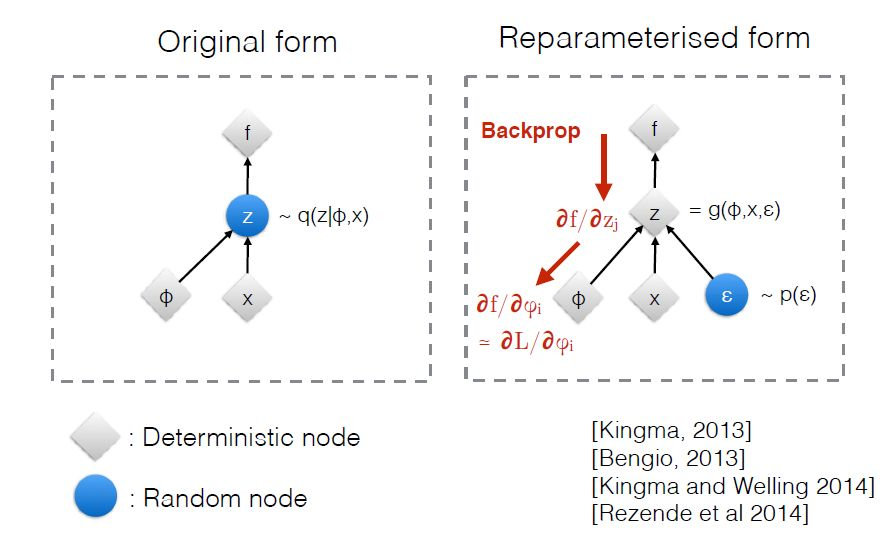If the sampling function $$g(\phi, x, \varepsilon)$$ was a normal distribution of some sort, without the randomness, the network could learn to set the variance to zero and propagate whatever value the mean is exactly. This might cause the network to take shortcuts and not learn latent variables. So, the e term gives a restriction to the encoder: you must encode in such a way that can still communicate sufficient information despite your output being purturbed in a very specific way. For the random normal case, the encoded has control of this purturbation by controlling $$\sigma$$, however, the encoded is penalized as $$\sigma$$ gets further from the value 1.0 (to match a standard normal). I wonder what would happen if you didn't let the encoder produce $$\sigma$$ at all and had it hard-coded to 1.0?